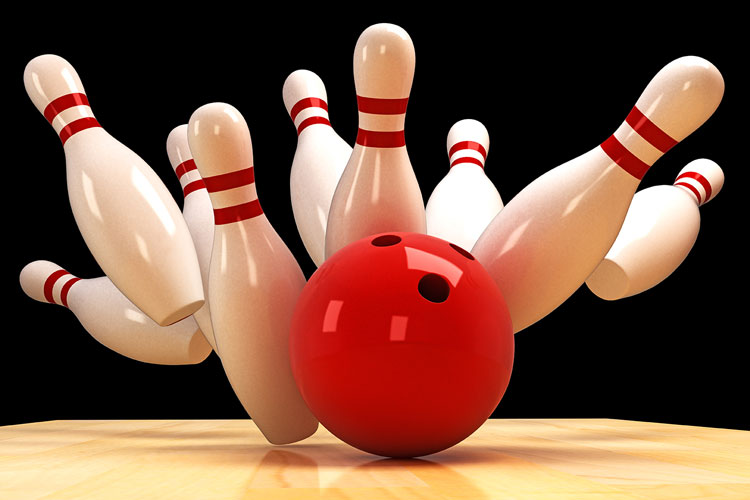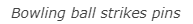STEMLabs V5
Momentum Alley Student

# Momentum# The Science of Momentum

Momentum can be thought of as the amount of motion that an object has. Momentum is determined by the object's mass (how much is moving) and its velocity (how fast it is moving). In physics, momentum is represented by "p" so the equation for determining momentum is p = mv (momentum equals mass times velocity). A heavier object, like a bowling ball, traveling at the same speed as a lighter object, like a kickball, would have greater momentum according to the laws of physics.

For this STEM Lab, you need to understand momentum and how it affects collisions because later, you will compete in a bowling game called the Strike Challenge. You will program the Speedbot to run into a ball in order to knock down pins and earn the highest score. Let's think about what happens when two objects collide. They transfer kinetic energy, yes. But, the momentum of each of the two colliding objects before the collision can predict how the two objects will react after the collision. We will return to this idea later.